# 基于新冠肺炎疫情下的防控策略优化Optimization of Prevention and Control Strategies Based on the COVID-19

DOI: 10.12677/MOS.2021.101021, PDF, HTML, XML, 下载: 61  浏览: 445  科研立项经费支持

Abstract: In 2019, the sudden new crown pneumonia epidemic (COVID-19) swept the world. This paper uses Matlab to establish an SEIR model and a quadratic exponential smoothing model to predict the number of confirmed cases and gross domestic product (GDP) in some typical countries, and con-siders the prevention and control strategy of the COVID-19 epidemic. The fuzzy evaluation model is used to forecast and analyze the number of newly confirmed cases.

1. 引言

2. 确诊病例的预测模型

2.1. 数据来源

1) 对货物贸易(除医疗物资外)采取措施；

2) 对医疗物资贸易采取措施；

3) 对船舶、航班、列车采取措施；

4) 对边境口岸采取措施；

5) 对人员入境采取措施；

6) 宣布进入紧急状态的国家(地区)。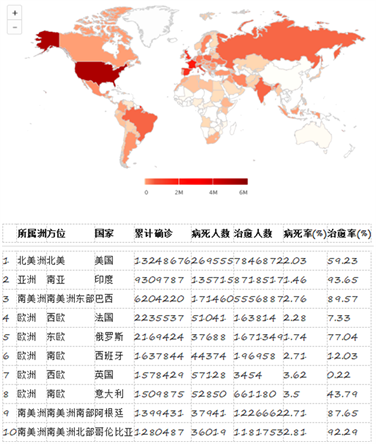Figure 1. As of November 27, 2020, the top ten countries (or regions) with existing confirmed cases of new crown pneumonia

2.2. 模型建立与分析

$\left\{\begin{array}{l}\frac{\text{d}S}{\text{d}t}=-\beta \left(I+\theta A\right)-pS+\lambda Q,\\ \frac{\text{d}Q}{\text{d}t}=pS-\lambda Q,\\ \frac{\text{d}E}{\text{d}t}=\beta \left(I+\theta A\right)-\sigma E,\\ \frac{\text{d}A}{\text{d}t}=\sigma \left(1-\rho \right)E-{\epsilon }_{A}A-{\gamma }_{A}A,\\ \frac{\text{d}I}{\text{d}t}=\sigma \rho E-{\gamma }_{I}I-{d}_{I}I-{\epsilon }_{I}I,\\ \frac{\text{d}D}{\text{d}t}={\epsilon }_{A}A+{\epsilon }_{I}I-{d}_{D}D-{\gamma }_{D}D,\\ \frac{\text{d}D}{\text{d}t}={\epsilon }_{A}A+{\epsilon }_{I}I+{\gamma }_{D}D.\end{array}$ (1)

1) S(t)——易感人群数量；

2) Q(t)——隔离人群数量；

3) E(t)——暴露人群数量；

4) A(t)——无症状感染人群数量；

5) I(t)——有症状感染人群数量；

6) D(t)——确诊人群数量；

7) R(t)——康复人群数量；

8) $\beta$ ——接触率；

9) $\rho$ ——隔离人群的隔离率；

10) $\lambda$ ——隔离人群的释放率；

11) $\sigma$ ——暴露人群转换为感染人群的转换率；

12) $\epsilon$ ——诊断率；

13) $1/\gamma$ ——人群康复率；

14) 1/d——人群死亡率。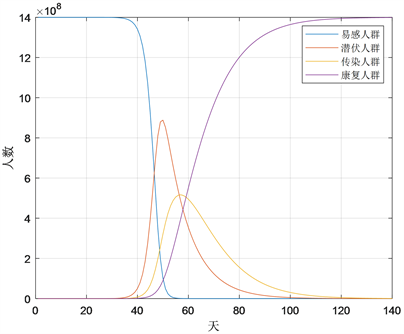Figure 2. Matlab simulation map

$R=\left(1+{}_{r}T{}_{L}\right)\cdot \left(1+{}_{r}T{}_{I}\right)$ (2)

$b\left(t\right)=b\left(t-\Delta t\right){\text{e}}^{r\Delta t}$ (3)

TLTI都是来自对现实情况的观察，TL表示被感染者的潜伏期的平均长度， ${T}_{L}={S}_{I}-{T}_{I}$ 、SI的定义分别为一个零感染者被感染的时间和他的下一个人被感染的时间的间隔。跟据参考文献  中，得到SITL分别为14天和7天。Table 1. Average value of basic reproduction number R

3. 疫情之下的经济预测趋势

3.1. 指数平滑模型

$\left\{\begin{array}{l}{S}_{t}^{\left(1\right)}=\alpha {y}_{t}+\left(1-\alpha \right){S}_{t-1}^{\left(1\right)}\\ {S}_{t}^{\left(2\right)}=\alpha {S}_{t}^{\left(1\right)}+\left(1-\alpha \right){S}_{t-1}^{\left(2\right)}\end{array}$ (4)

${\stackrel{^}{y}}_{t+m}={a}_{t}+{b}_{t}m,m=1,2,\cdot \cdot \cdot ,$ (5)

$\left\{\begin{array}{l}{a}_{t}=2{S}_{t}^{\left(1\right)}-{S}_{t}^{\left(2\right)},\\ {b}_{t}=\frac{\alpha }{1-\alpha }\left({S}_{t}^{\left(1\right)}-{S}_{t}^{\left(2\right)}\right),\end{array}$ (6)

3.2. 解题过程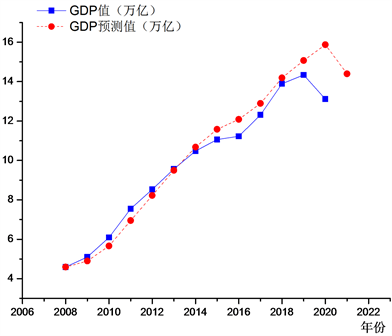Figure 3. Chinese GDP compared with the predicted values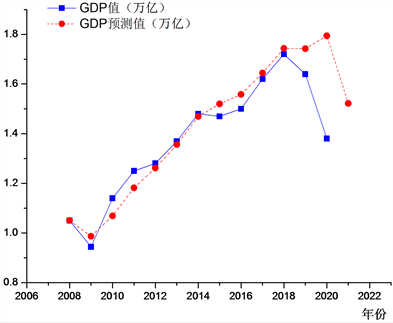Figure 4. Korean GDP compared with the predicted values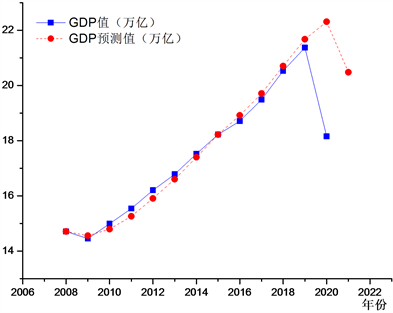Figure 5. American GDP compared with the predicted values

3.3. 模型分析

4. 新增确诊病例的预测模型

4.1. 建立模型

$U=\left\{{u}_{1},{u}_{2},{u}_{3},\cdots ,{u}_{n}\right\}.$ (7)

$V={\left\{v}_{1},{v}_{2},\cdots ,{v}_{m}\right\}.$ (8)

$A=\left[{a}_{1},{a}_{2},\cdots ,{a}_{n}\right].$ (9)

${R}_{i}=\left[{r}_{i1},{r}_{i2},\cdots ,{r}_{in}\right].$ (10)

$R=\left[\begin{array}{cccc}{r}_{11}& {r}_{12}& \cdots & {r}_{1m}\\ {r}_{21}& {r}_{22}& \cdots & {r}_{2m}\\ ⋮& ⋮& \ddots & ⋮\\ {r}_{n1}& {r}_{n2}& \cdots & {r}_{nm}\end{array}\right],$ (11)

4.2. 数据分析Figure 6. The Matlab forecast analysis chart of the trend of newly diagnosed people in Korea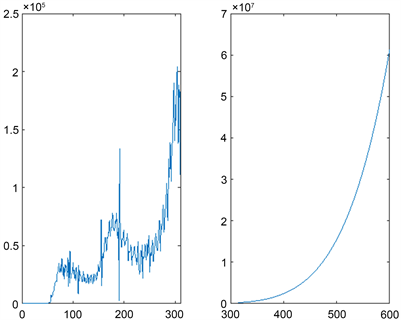Figure 7. The Matlab forecast analysis chart of the trend of newly diagnosed people in American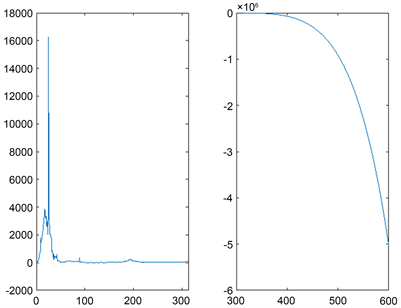Figure 8. The Matlab forecast analysis chart of the trend of newly diagnosed people in China

5. 结语

NOTES

*通讯作者。

  湖南省第五届研究生数学建模竞赛论文提交说明[EQ/OL]. http://sxtj.hutb.edu.cn/column/sxtjxy_shiyi/2020/1606392094439.shtml, 2021-1-17.  新型冠状病毒肺炎[EQ/OL]. https://h5.baike.qq.com/mobile/overview_detail.html?name=%E6%96%B0%E5%9E%8B%E5%86%A0%E7%8A%B 6%E7%97%85%E6%AF%92%E8%82%BA%E7%82%8E&adtag=sg.bk.bing.rp &VNK=612dfa3a&tab=gaishu&TNK=0016e0c3#gaishu, 2020-11-28.  新冠肺炎疫情实时动态[EQ/OL]. https://sa.sogou.com/new-weball/page/sgs/epidemic?type_page=VR, 2020-11-28.  佟家栋, 盛斌, 蒋殿春, 严兵, 戴金平, 刘程. 新冠肺炎疫情冲击下的全球经济与对中国的挑战[J]. 国际经济评论, 2020(3): 9-28+4.  何枭吟, 王晗, 焦成焕. 新冠肺炎疫情对全球经济的影响及中国对策[J]. 重庆三峡学院学报, 2020, 36(6): 65-72.  连俊. 只有有效控制疫情才能实现经济增长[N]. 经济日报, 2020-11-02(003).  丁尖. COVID-19防控措施的动力学模型分析与计算[D]: [硕士学位论文]. 长春: 吉林大学, 2020.  陈彬, 杨妹, 艾川, 马亮, 朱正秋, 陈海亮, 朱蒙娜, 许伟. 基于人工社会的疫情传播风险预测和防控措施评估[J/OL]. 系统仿真学报: 1-7[2020-11-30].  曹盛力, 冯沛华, 时朋朋. 修正SEIR传染病动力学模型应用于湖北省2019冠状病毒病(COVID-19)疫情预测和评估[J]. 浙江大学学报(医学版), 2020, 49(2): 178-184.  曹媛媛. 网络消费动态价格指数的二次平滑处理与预测[J]. 统计与决策, 2019, 35(17): 5-9.  武经纬. 基于多级模糊综合评价模型的健康水平评估与测控[J]. 科学咨询(科技•管理), 2020(8): 78-79.  曹可, 吕继续. 基于最小二乘法预测传染病的发病人数[J]. 科技资讯, 2019, 17(33): 228-229.